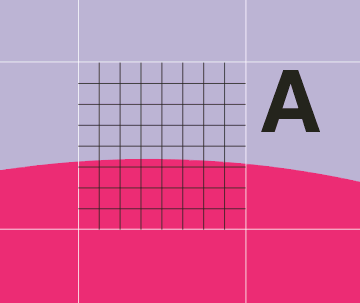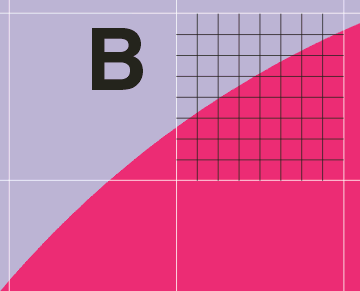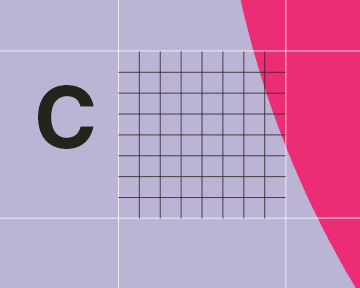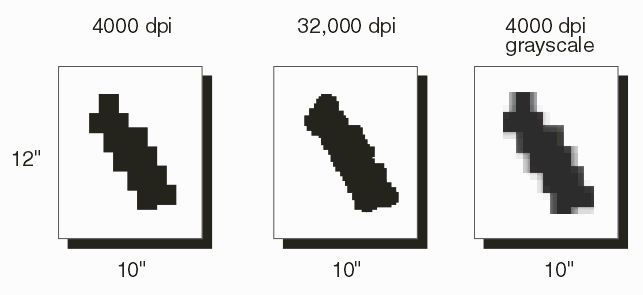## GrayScale Output from Super SamplingFigure 4: Pixel A is supersampled 8X giving 64 levels of gray

If the Gerber vector data is rasterized at 8X of the final resolution each final pixel is composed of 64 mini pixels.

The program counts how many of the "mini" pixels are inside the edge. In this example one could definitively say that 24 of the 64 pixels are completely inside. (The center point of the pixel is used to determine whether or not it is considered "on")

Pixel A would be assigned a value of 96 in the final gray scale (8 bit) output.Figure 5: Detail of Pixel B

Pixel B -- 40 of the mini pixels have their center point inside of the trace.

Therefore pixel B would be assigned a gray scale value of 40/64 or in 8 bit range a value of 160.Figure 6: Detail of Pixel C

For pixel C, only 3 of the mini pixels are "on"

Pixel C would be assigned a value of 12 in the 8 bit grayscale output.

## Computational Costs of Super Sampling

To get the extra information about the edge pixels it is necessary to rasterize the entire input file at some multiple of the final resolution (typically the multiple is 8X or 16X.) This means that the computation work (and associated memory requirements) goes up the by square of the super-sampling: for an 8X supersample there is 64 times increase and for a 16X supersampling there is a 256 times increase in processing time.Figure 7: Comparison of monochrome, super sample and gray scale

Consider a 10x12 inch image which requires 15 seconds to rasterize at 4000 dpi (monochrome.). To obtain a gray scale image at 4000 dpi using 8X supersampling will require a minimum of 225 seconds to rasterize plus addtional time to convert to grayscale.

Memory requirements are also greatly increased. The 4000 dpi monochrome image requires a raster buffer of:

```
10 inch x 12 inch x 4000 dots/inch x 4000 dots/inch
----------------------------------------------------   =   240 MBytes
8 pixels per byte

```

(Actually, 480 MBytes are needed since the raster process is double buffered.)

The 8x super sampling will require 64 X 480 MB or a total of 7.68 GB of RAM. Since this much RAM is not available, the GBR_RIP program must subdivide the image into small stripes and process each stripe individually; combining the results on disk. The more stripes required the more disk IO and disk space is used.

Prior to utilizing super sampling, the system engineer should do some back-of-the-envelope calculations to insure that his goals are consistent with the available computation time and hardware (i.e. a fast CPU, lots of RAM and lots of hard disk space)

Gray Scale       1   2   3